What is a logic gate and what is its type? - Electrical C & V

# Electrical C & V

electrical engineering information, basic electrical, electrical laws, machines, transformer, motors, types of motor, induction motor, dc motor, servo motor, synchronous motor, dynamo or generator, power system, transmission line, mcc, generation station, electronics, devices, diodes, sensors, circuit breaker, miniature circuit breaker, air circuit breaker, vaccum circuit breaker, sf6 circuit breaker, variable frequency drive

## 07 March 2020

Logic gates

Logic gates are the basic building blocks of any digital system. The logic gates are an electronic circuit. It has one or more inputs and only one output. The relationship between input and output is based on a particular logic.

In this, the input and output are in binary form i.e. the value of input / output in it will be only 0 (low) and 1 (high).

Types of logic Gates

Generally, there are three types of logic gates -

1. AND Gate
2. OR Gate
3. NOT Gate

There are many other types of logic gates which are at the base of these three logic gates. like :-
NAND GATE, NOR GATE, XOR GATE.

1. AND Gate

AND Gate has two or more inputs but output is only one. The output of AND Gate is high only when all inputs are high. AND Gate performs multiplication operation on two or more inputs. Display the operation of AND Gate with (.).

Ex: - X = A AND B
X = A.B

Logic diagram: - Here two inputs are A and B and one is output X = A.B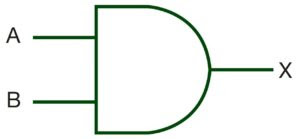Truth Table: -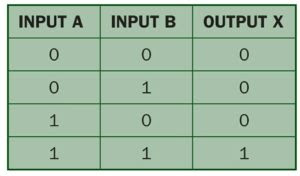2. OR GATE

OR GATE has two or more input signals and only one output signal. This is called OR GATE because the output is high when any one input or all inputs are high. In OR GATE, addition (s) operation is performed on two or more inputs. The OR GATE operation is displayed with the (+) symbol.

Ex: - X = A OR B
X = A + B

Logic diagram: -Truth Table: -3. NOT GATE

NOT GATE has only one input and one output because its output state is opposite to the input state. That is, the input is high when the output is low. NOT GATE is also called complement, negation or inverter. The NOT GATE operation is displayed with a bar or (¯) symbol.

Ex: - X = NOT A
X = A¯

Logic diagram: -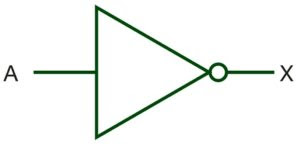Truth Table: -4. NAND GATE

Adding an AND GATE with NOT GATE generates a new GATE called NAND GATE. It performs NOT and AND operations. What is NAND GATE is the compliment (¯) of AND GATE.

Ex: - X = A NAND B
X = (AB) ¯

Logic diagram: -Truth Table: -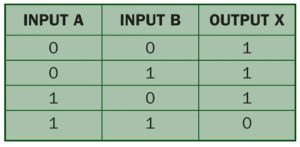5. NOR GATE

It is a combination of NOT and OR GATE. The GATE received by adding a NOT GATE and an OR GATE is called NOR GATE. What is NOR GATE is a compliment to OR GATE.

Ex: - X = A NOR B
X = (A + B) ¯

Logic diagram: -Truth Table: -6. XOR GATE

XOR GATE is widely used in digital circuits although it is not a basic operation but can be constructed using basic GATES (AND, OR, NOT) or universal GATE (NAND or NOR).

XOR can be used in half adder, full adder, and substractor. XOR is also known as Ex-OR.

Logic diagram: -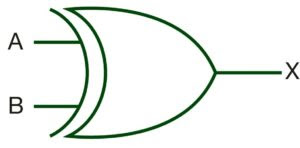Truth Table: -1.2.